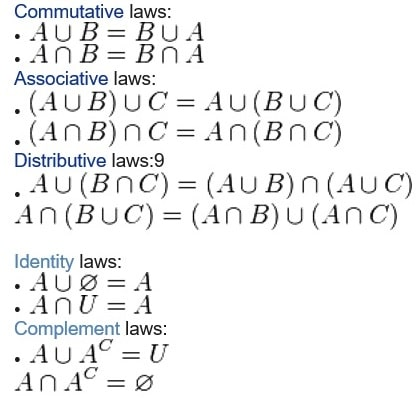# Set theory question

{`1) If P={distinct letters in the word college} and T={distinct letters in the word algebra}, what is |PxT|?`}

2) Given the following LAWS of Set Theory, use them to prove a and b below.1. Prove that A ⋃ ( A ⋂ B) = A
2. Prove that (A ⋃ B)’ = (A’ ⋃ B’)’

3) Two countably infinite sets are considered to have equal cardinality if and only if there exists a one-to-one and onto function that maps them from one to another. Use this fact to show that the following sets have the same cardinality.

{`S1 = { x | x is an integer } S2 = { y | y is an even integer }`}

4) If |S| = 5 and |R| = 4 then what is |SxR|?

5) What is the power set of the set of all letters in the word “babble” ?

6) Given two sets S and R such that |S| = M and |R| = N what is the minimum and maximum cardinality of the following?

1. S ⋃ R
2. S ⋂ R
3. S x R
4. 2S

7) Write a simple definition for the set of all positive integers which are a multiple of 12.

8) If our Universal set is the set of all integers, and S is the set you defined in question #7, write a definition for the set ( U – S ) ( the set of elements in U but not in S.

9) Show that the U defined in question #8 has the same cardinality as the set (U – S) you defined in question #8.